# Pre-Algebra : Order of Operations

## Example Questions

← Previous 1 3 4 5 6 7 8 9 12 13

### Example Question #1 : Order Of Operations

Simplify the expression below.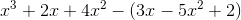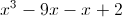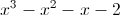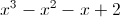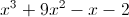Explanation:

First, distribute the negative sign into the parentheses.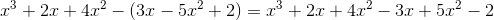Next, combine like terms.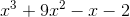Note that all operations in this problem are addition and subtraction; there is no need to FOIL or multiply.

### Example Question #2 : Operations

Solve the following problem: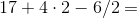Explanation:

First, work from left to right completing multiplication and division, then work from left to right completing addition and subtraction.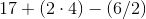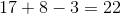### Example Question #1 : Operations And Properties

Suppose you know the value of, and you want to evaluate the expression: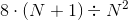In which order would you carry out the four operations in the expression?

Add, square, multiply, divide

Add, square, divide, multiply

Multiply, divide, add, square

Multiply, add, divide, square

Add, multiply, divide, square

Add, square, multiply, divide

Explanation:

By order of operations, always carry out any operations within parentheses first; this is the addition.  This removes the parentheses; what remains is a square, a multiplication, and a division. Since there are no more grouping symbols, square next. The multiplication is done next, as multiplications and divisions are performed in left-to-right order.

In summary: Add, square, multiply, divide

### Example Question #1 : Operations And Properties

Suppose you know the value of, and you want to evaluate the expression: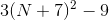In which order would you carry out the four operations in the expression?

Square, multiply, add, subtract

Multiply, add, square, subtract

Square, add, multiply, subtract

Add, square, multiply, subtract

Multiply, square, add, subtract

Add, square, multiply, subtract

Explanation:

By order of operations, always carry out any operations within parentheses first; this is the addition. This removes the parentheses; what remains is a square, a multiplication, and a subtraction. This is the correct order in the absence of grouping symbols.

In summary: Add, square, multiply, subtract

### Example Question #1 : Operations And Properties

Simplify the expression.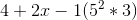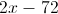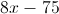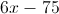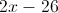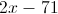Explanation:

The order of operations is parenthesis, exponents, multiplication, division, addition, subtraction (PEMDAS).First, we will evaluate the parentheses. Within the parentheses, we need to solve the exponent, then multiply,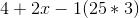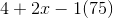Now that the parenthesis is evaluated, we need to multiply.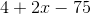Finally, we add and subtract. We can arrange the terms in any order.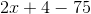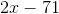### Example Question #6 : Operations

Simplify: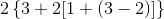Explanation:

Follow the order of operations: parentheses, exponents, multiplication, division, addition, and subtraction. Work from left to right, and start with the innermost nested operations when dealing with multiple parentheses: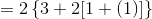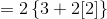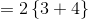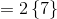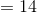### Example Question #1 : Order Of Operations

Simplify: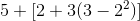Explanation:

Follow the order of operations: parentheses, exponents, multiplication, division, addition, and subtraction. Work from left to right, and start with the innermost nested operations when dealing with multiple parentheses: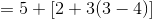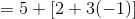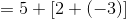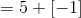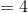### Example Question #1 : Operations

Simplify: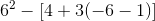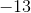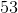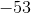Explanation:

Follow the order of operations: parentheses, exponents, multiplication, division, addition, and subtraction. Work from left to right, and start with the innermost nested operations when dealing with multiple parentheses: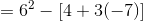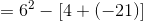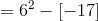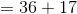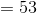### Example Question #1 : Operations And Properties

Simplify: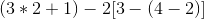Explanation:

Follow the order of operations: parentheses, exponents, multiplication, division, addition, and subtraction. Work from left to right, and start with the innermost nested operations when dealing with multiple parentheses: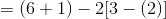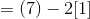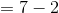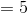### Example Question #2 : Order Of Operations

Simplify: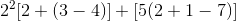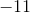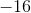Explanation:

Follow the order of operations: parentheses, exponents, multiplication, division, addition, and subtraction. Work from left to right, and start with the innermost nested operations when dealing with multiple parentheses: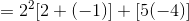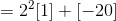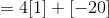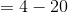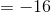← Previous 1 3 4 5 6 7 8 9 12 13

### All Pre-Algebra Resources